Reasoning Question: Seating Arrangement(Based on IBPS Clerk Mains 2018) Set 67

Welcome to Online Reasoning Section with explanation in AffairsCloud.com. Here we are creating Best question samples from Puzzle with explanation, which is common for competitive exams. We have included Some questions that are asked in previous exams !!!

(Direction 1 – 5): Study the following information carefully to answer the given questions:

Ten persons A, B, C, D, E F, G, H, I and J are standing in a queue facing north. Their daily income is of 2000, 3000, 5000, 7000 and 10000. Three persons stand between G and B. G stands behind B. B stands either at the beginning or at the end. E stands adjacent to G. Three persons stand between E and I. As many persons stand before E as stand after J. Five persons stand between D and H. H stand behind D. F stand behind A. F does not stand at extreme end.
M * N means M receive more income than N.
M & N means M receive less income than N.
M % N means M receives same cash as N.
D%H, H*C, C*B, B%E%G, G&C%I, I*A*J%F.
Note: Not more than 3 persons receives same amount of income.

1. What is the total income received by E, A and F?
1) 20000
2) 10000
3) 12000
4) 14000
5) None of these
Solution:
Persons – A, B, C, D, E F, G, H, I and J
Income – 2000, 3000, 5000, 7000 and 10000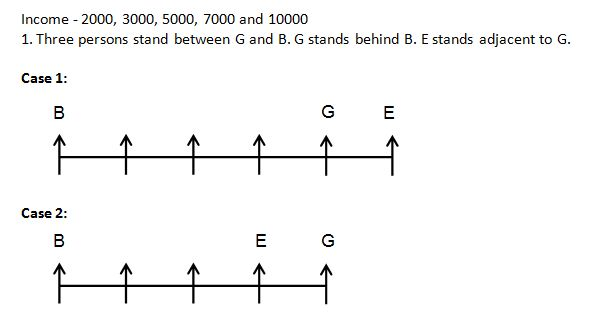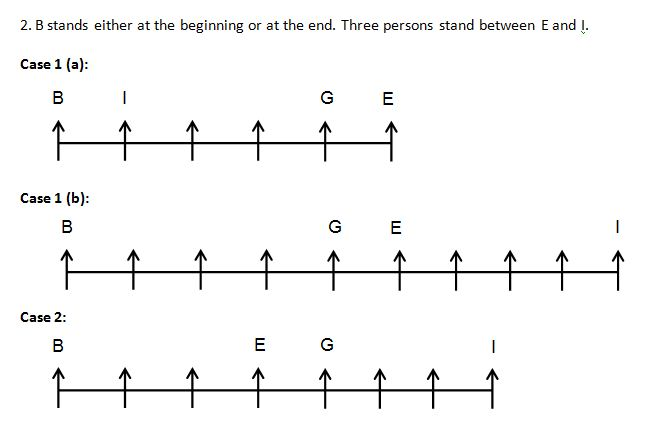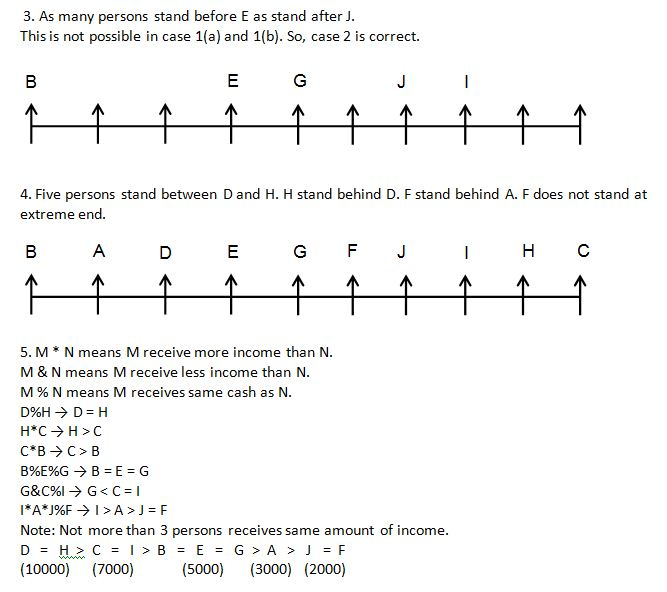2. What is the difference between the income of C and J?
1) 4000
2) 5000
3) 3000
4) 8000
5) None of these

3. Who among the following sits at the extreme end?
1) C
2) H
3) G
4) E
5) None of these

4. Who among the following receives the highest income?
1) F
2) H
3) I
3) G
5) A

5. Who among the following receives the amount of income alone?
1) H
2) D
3) A
4) F
5) None of these

(Directions 6 – 10): Read the following information carefully and answer the questions given below it:

Sixteen persons A, B, C, D, E, F, G, H, M, N, O, P, Q, R, S and T are sitting around two circular tables. There is small circular table inside the bigger circular table. The persons sitting around the inner circular table faces outside and the persons sitting around the outer circular table faces inside in such a way persons sitting on both tables facing each other.

C sits to the immediate right of F. Only two persons are sitting between C and G who faces O. G and O sit on different tables. Two persons sit between B and M. Four persons are sitting between D and F. H sits to the immediate left of G and faces Q. F who faces P faces outside. A faces C. The one who faces E sits exactly between N and T who faces M. Three persons are sitting between O and R who sits exactly between A and E.

1. Four of the following are alike in a certain way and form a group. Which of the following does not belong to that group?
1) S
2) H
3) A
4) T
5) N
Solution:
Persons – A, B, C, D, E, F, G, H, M, N, O, P, Q, R, S and T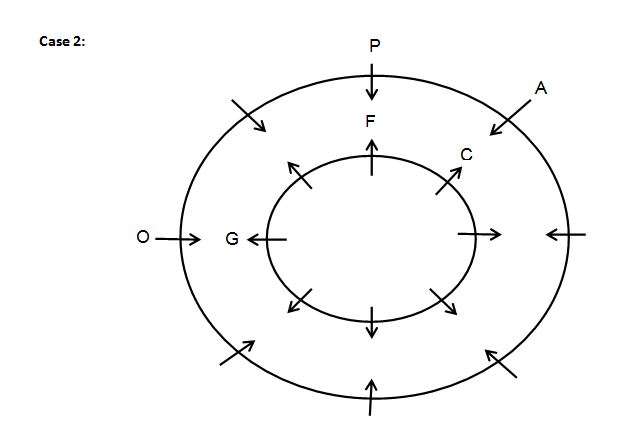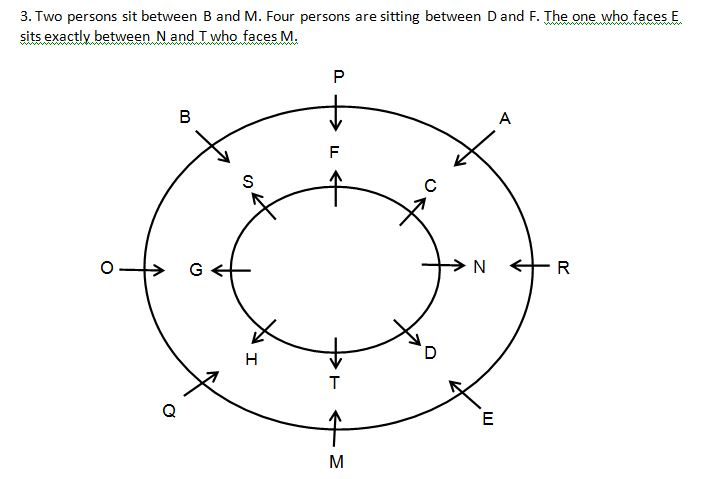2. Who among the following faces S?
1) Q
2) O
3) A
4) G
5) B

3. Who among the following sits third to the right of N?
1) H
2) Q
3) S
4) T
5) F

4. Who among the following is the immediate neighbor of Q?
1) H
2) M
3) E
4) B
5) R